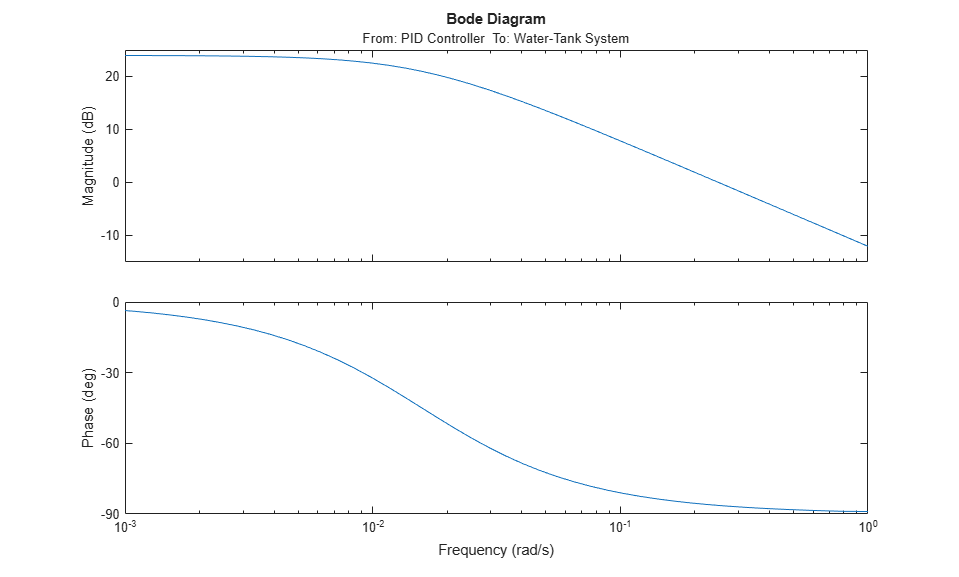This example shows how to programmatically linearize a watertank Simulink Model feedback control system. In the example, you obtain an open-loop linearized model of the water-tank system at an operating point where the tank level is at a steady state.

For more information on programmatically specifying input and output points for linearizing a model, see Specify Portion of Model to Linearize and Specify Portion of Model to Linearize at Command Line.

For more information on finding operating points for linearization, see Compute Steady-State Operating Points from Specifications and Compute Operating Points from Specifications at the Command Line.

Open the model.

mdl = 'watertank';
open_system(mdl)In this model, there is an expected steady-state operating condition when the water level is at H = 10.

Compute Operating Point

To linearize a model, you must first obtain an operating point for the conditions at which you want to linearize the model. One approach is to first simulate the model and extract an operating point when the simulation is near the desired value. You can then use this operating point as a starting point for an optimization-based search (trimming) for a steady-state operating point.

Using the findop function, simulate the model and obtain an operating point using the model conditions after 10 seconds.

opsim = findop(mdl,10)
opsim =

Operating point for the Model watertank.
(Time-Varying Components Evaluated at time t=10)

States:
----------
<strong>x</strong>
<strong>______</strong>

(1.) watertank/PID Controller/Integrator/Continuous/Integrator
1.6949
(2.) watertank/Water-Tank System/H
10.08

Inputs: None
----------

In this operating point, H is not at the desired value of 10. However, you can use this operating point to initialize a search for an operating point where H = 10.

To configure the operating point search, first create an operating point specification object.

opspec = operspec(mdl);

Initialize the values of the states in the operating point specification with the state values in the operating point opsim.

opspec = initopspec(opspec,opsim);

Trim the model using the operating point specifications.

opss = findop(mdl,opspec);
Operating point search report:
---------------------------------

opreport =

Operating point search report for the Model watertank.
(Time-Varying Components Evaluated at time t=10)

Operating point specifications were successfully met.
States:
----------
<strong>Min</strong>       <strong>x</strong>       <strong>Max</strong>    <strong>dxMin</strong>        <strong>dx</strong>         <strong>dxMax</strong>
<strong>____</strong>    <strong>______</strong>    <strong>___</strong>    <strong>_____</strong>    <strong>___________</strong>    <strong>_____</strong>

(1.) watertank/PID Controller/Integrator/Continuous/Integrator
-Inf    1.2649    Inf      0                0      0
(2.) watertank/Water-Tank System/H
0        10    Inf      0      -1.0991e-14      0

Inputs: None
----------

Outputs: None
----------

In this operating point, H = 10 as expected. The operating point is at a steady state since the dx values for the model states are near zero.

Configure Linear Analysis Points

To linearize the model, you must specify the portion of the model that you want to linearize. Linear analysis points specify the inputs and outputs of a linearized model. To extract the open loop linearized model of the water-tank plant, add an input point at the output of the Controller block and an output point, with a loop opening, at the output of the Water-Tank System block.

Specify the input point.

watertank_io(1) = linio('watertank/PID Controller',1,'input');

Specify the output point with a loop opening.

watertank_io(2) = linio('watertank/Water-Tank System',1,'openoutput');

Linearize and Analyze Model

You can now linearize the model using the specified operating point and linear analysis points.

sys = linearize(mdl,opss,watertank_io);

The resulting model is a state-space object that you can analyze using any of the tools in the Control System Toolbox™ software. For example, view the frequency response of the linear model.

bode(sys)bdclose(mdl)